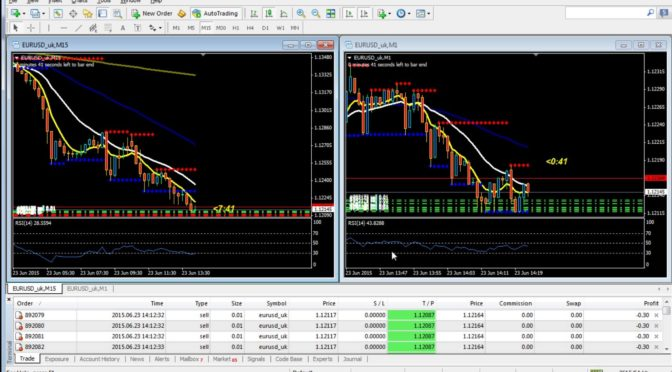--10 views

# What is a Pip?Basic terms in Forex Trading are important to understand

There are a lot of forex terms that you will encounter when starting to enter trading in the forex market, one of the terms that you will definitely hear is pip.This time we will discuss completely and thoroughly related to the term in the forex market.

This term is very important for you to understand as the basis before entering and start trading in the forex market.

Here’s more explanation for you all.

## What is a Pip?

Pip is an abbreviation of Price Interest Point sentence. The term refers to units of units used to measure exchange rate changes between 2 currencies.

The currency of the country whose value is displayed into 4 decimal places means that in 1 pip is equal to 0.0001. Let’s take a simple example, if the EUR / USD currency pair from the previous price of 1.1300 then rises to 1.1305, then it means there is a 5 pips increase in the currency pair.

Likewise with the currency whose value is displayed into 2 decimal places, then in 1 pip equals 0.01. For example like this the USD / JPY currency pair from the 100.01 price rose to 100.05, then there is a 4 pip increase in it.In forex trading practice, you will find currencies written in 4 decimal places, except for currency pairs related to Japanese Yen.

In addition to the above one, there is also a forex broker that presents currency pairs in the form of 5 decimal places, in this case JPY currency pair has 3 decimal places. If this happens, then you need to be more vigilant because the last digit number is not pip, but pipettes. Make no mistake in measuring price movements, because 1 pipettes is equal to 1/10 pip. For example like this, the USD / JPY currency pair from 100.059 drops to 100,015, when this condition occurs it means there is a decrease of 4.4 pip instead of 44 pips.

### Another Example In Pips Calculations

For example, let’s just say the USD / CAD exchange rate is equal to 1.0200 in this case 1 USD is equal to 1,0200 CAD.

How to know the pip value of the currency pair?

The trick is to multiply the value of the change in Counter Currency with the ratio of the existing exchange rate. Thus, [.0001 x (1 USD / 1,0200 CAD)] this calculation will yield 0.00009804 per transaction unit.

From the above calculation, meaning that when we make a transaction of 10,000 units USD / CAD, then there will be a change in exchange rate of 1 pip which is equivalent to 0.98 USD. The value is obtained by 10,000 units multiplied by 0.0000984 USD / unit.

The next thing you need to understand is the global forex market and it means that not everyone uses the same base currency with the account you use.Curiosity will arise with the value of pip if measured in the currency of the account. If you want to answer this question, then you need to equalize each pip into the currency you use in your account.

The trick is not too difficult to do, because you only need to multiply or divide the Pip Value with the exchange rate of currency in the account with the currency you use.

We’ll use the GBP / JPY currency pair, let’s say we’ll convert the .813 pip value in GBP to USD. The exchange rate used is GBP / USD 1.5590.The calculations are as below:

.813 GBP per pip / (1 GBP / 1.5590 USD) Or

[(.813 GBP) / (1 GBP)] x (1.5590 USD) = 1.2674 USD for each 1 pip move.

Through the above calculation, we can know every movement of .01 pip in the GBP / JPY pair, the value of 10,000 units is equivalent to 1.27 USD.

Then what if the currency to be searched pip value is the Base Currency of the exchange rate. The way it can be done is to multiply the value of pip by the exchange rate ratio.

Let’s say the USD / CAD pair and we want to find the pip value of .98 USD in New Zealand Dollar using .7900 as the exchange rate comparison.

The calculations are like this:

0.98 USD per pip x (1 NZD / .7900 USD) Or

[(0.98 USD) / (.7900 USD)] x (1 NZD) = 1.2405 NZD

Thus in every 100 pips movement in the USD / CAD pair, the value of 10,000 units is equal to or equal to 1.24 NZD.

Understand well the various examples and basic notions as above make you more familiar with the basic forex that needs to be owned by a trader. Understanding the basic forex is very important for you who are serious to trade in the forex market. Through a good understanding of the basic forex, then you can easily learn the advanced techniques related to the existing forex trading.

In addition to knowing the term pip in the forex world and understand how to calculate the value of pip, then you can get a clear picture in determining the value of transactions that occur in the trading being done. You also need to understand various other terms in the world of forex such as Leverage, Spread, and so forth. We have created our own articles regarding the forex terms mentioned above.

We hope that the information presented above can provide many benefits to all of you who are just starting to invest in the forex market. Do not forget also to share the above information to others through social media you have. So that more and more people know the basic terms that exist in forex.

If you have any further questions regarding the discussion we have mentioned above. Feel free to inquire through the comments field provided below. We will be happy to answer any incoming questions.

Hopefully the above information can inspire you all.